## mlcourse.ai – Open Machine Learning Course¶

Authors: Vitaliy Radchenko, and Yury Kashnitsky. Translated and edited by Christina Butsko, Egor Polusmak, Anastasia Manokhina, Anna Shirshova, and Yuanyuan Pao. This material is subject to the terms and conditions of the Creative Commons CC BY-NC-SA 4.0 license. Free use is permitted for any non-commercial purpose.

# Topic 5. Ensembles and random forest¶

## Article outline¶

$\DeclareMathOperator{\Var}{Var}$ $\DeclareMathOperator{\Cov}{Cov}$ $\DeclareMathOperator{\Corr}{Corr}$ $\DeclareMathOperator{\Err}{Err}$ $\DeclareMathOperator{\Bias}{Bias}$ $\DeclareMathOperator{\E}{\mathbb{E}}$

Leo Breiman managed to apply bootstrapping not only in statistics but also in machine learning. He, along with Adel Cutler, extended and improved the random forest algorithm proposed by Tin Kam Ho. They combined the construction of uncorrelated trees using CART, bagging, and the random subspace method.

Decision trees are a good choice for the base classifier in bagging because they are quite sophisticated and can achieve zero classification error on any sample. The random subspace method reduces the correlation between the trees and thus prevents overfitting. With bagging, the base algorithms are trained on different random subsets of the original feature set.

The following algorithm constructs an ensemble of models using the random subspace method:

1. Let the number of instances be equal to $\large \ell$, and the number of features be equal to $\large d$.
2. Choose $\large L$ as the number of individual models in the ensemble.
3. For each model $\large l$, choose the number of features $\large dl < d$. As a rule, the same value of $\large dl$ is used for all the models.
4. For each model $\large l$, create a training set by selecting $\large dl$ features at random from the whole set of $\large d$ features.
5. Train each model.
6. Apply the resulting ensemble model to a new instance by combining the results from all the models in $\large L$. You can use either majority voting or aggregation of the posterior probabilities.

## 1. Algorithm¶

The algorithm for constructing a random forest of $\large N$ trees goes as follows:

• For each $\large k = 1, \dots, N$:

• Generate a bootstrap sample $\large X_k$.
• Build a decision tree $\large b_k$ on the sample $\large X_k$:

• Pick the best feature according to the given criteria. Split the sample by this feature to create a new tree level. Repeat this procedure until the sample is exhausted.
• Building the tree until any of its leaves contains no more than $\large n_\text{min}$ instances or until a certain depth is reached.
• For each split, we first randomly pick $\large m$ features from the $\large d$ original ones and then search for the next best split only among the subset.

The final classifier is defined by: $$\large a(x) = \frac{1}{N}\sum_{k = 1}^N b_k(x)$$

We use the majority voting for classification and the mean for regression.

For classification problems, it is advisable to set $\large m = \sqrt{d}$. For regression problems, we usually take $\large m = \frac{d}{3}$, where $\large d$ is the number of features. It is recommended to build each tree until all of its leaves contain only $\large n_\text{min} = 1$ examples for classification and $\large n_\text{min} = 5$ examples for regression.

You can see random forest as bagging of decision trees with the modification of selecting a random subset of features at each split.

## 2. Comparison with Decision Trees and Bagging¶

In :
# Disable warnings in Anaconda
import warnings
import numpy as np
warnings.filterwarnings('ignore')

%matplotlib inline
from matplotlib import pyplot as plt
plt.style.use('ggplot')
plt.rcParams['figure.figsize'] = 10, 6
%config InlineBackend.figure_format = 'retina'

import seaborn as sns
from sklearn.ensemble import RandomForestRegressor, RandomForestClassifier
from sklearn.ensemble import BaggingClassifier, BaggingRegressor
from sklearn.tree import DecisionTreeRegressor, DecisionTreeClassifier
from sklearn.datasets import make_circles
from sklearn.model_selection import train_test_split

In :
n_train = 150
n_test = 1000
noise = 0.1

# Generate data
def f(x):
x = x.ravel()
return np.exp(-x ** 2) + 1.5 * np.exp(-(x - 2) ** 2)

def generate(n_samples, noise):
X = np.random.rand(n_samples) * 10 - 5
X = np.sort(X).ravel()
y = np.exp(-X ** 2) + 1.5 * np.exp(-(X - 2) ** 2)\
+ np.random.normal(0.0, noise, n_samples)
X = X.reshape((n_samples, 1))

return X, y

X_train, y_train = generate(n_samples=n_train, noise=noise)
X_test, y_test = generate(n_samples=n_test, noise=noise)

# One decision tree regressor
dtree = DecisionTreeRegressor().fit(X_train, y_train)
d_predict = dtree.predict(X_test)

plt.figure(figsize=(10, 6))
plt.plot(X_test, f(X_test), "b")
plt.scatter(X_train, y_train, c="b", s=20)
plt.plot(X_test, d_predict, "g", lw=2)
plt.xlim([-5, 5])
plt.title("Decision tree, MSE = %.2f"
% np.sum((y_test - d_predict) ** 2))

# Bagging with a decision tree regressor
bdt = BaggingRegressor(DecisionTreeRegressor()).fit(X_train, y_train)
bdt_predict = bdt.predict(X_test)

plt.figure(figsize=(10, 6))
plt.plot(X_test, f(X_test), "b")
plt.scatter(X_train, y_train, c="b", s=20)
plt.plot(X_test, bdt_predict, "y", lw=2)
plt.xlim([-5, 5])
plt.title("Bagging for decision trees, MSE = %.2f" % np.sum((y_test - bdt_predict) ** 2));

# Random Forest
rf = RandomForestRegressor(n_estimators=10).fit(X_train, y_train)
rf_predict = rf.predict(X_test)

plt.figure(figsize=(10, 6))
plt.plot(X_test, f(X_test), "b")
plt.scatter(X_train, y_train, c="b", s=20)
plt.plot(X_test, rf_predict, "r", lw=2)
plt.xlim([-5, 5])
plt.title("Random forest, MSE = %.2f" % np.sum((y_test - rf_predict) ** 2));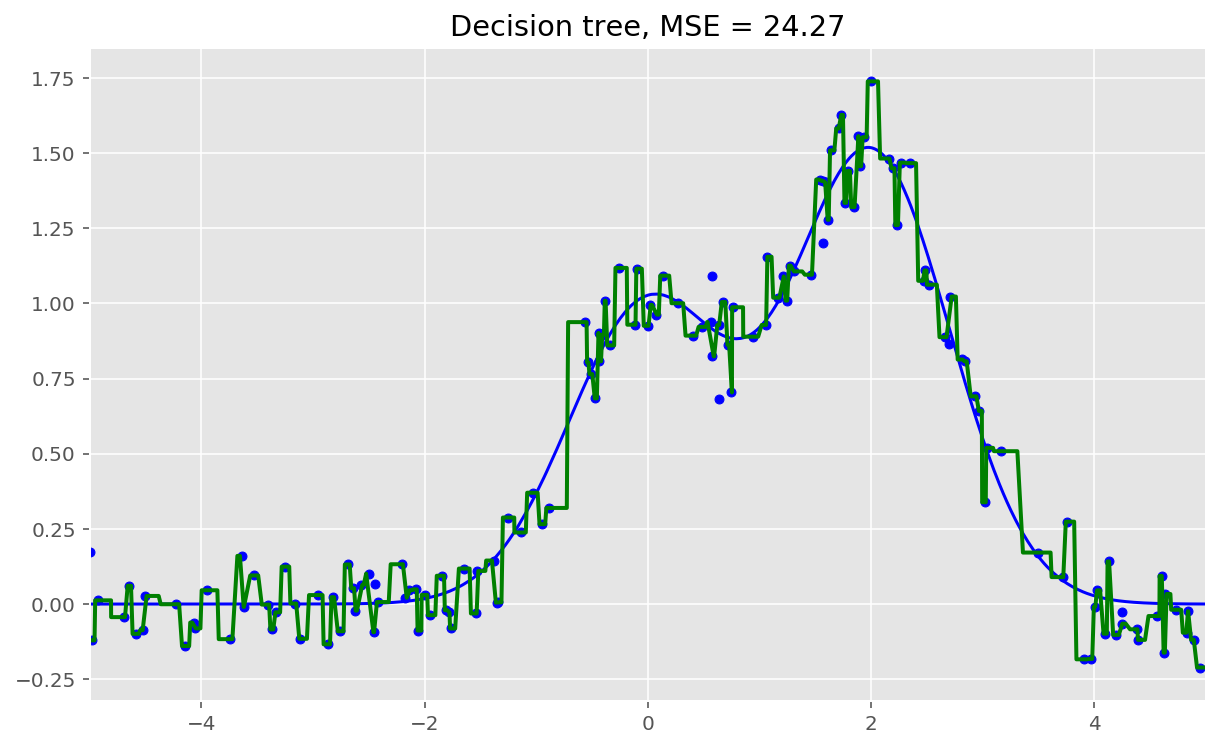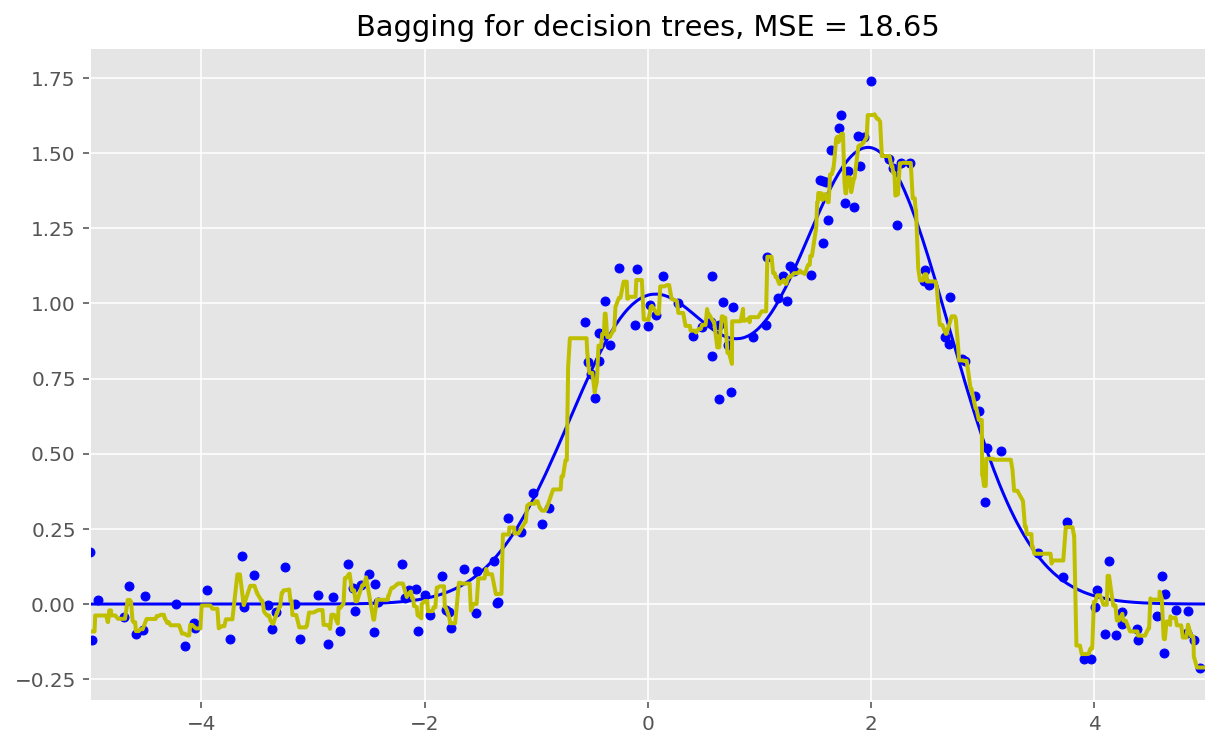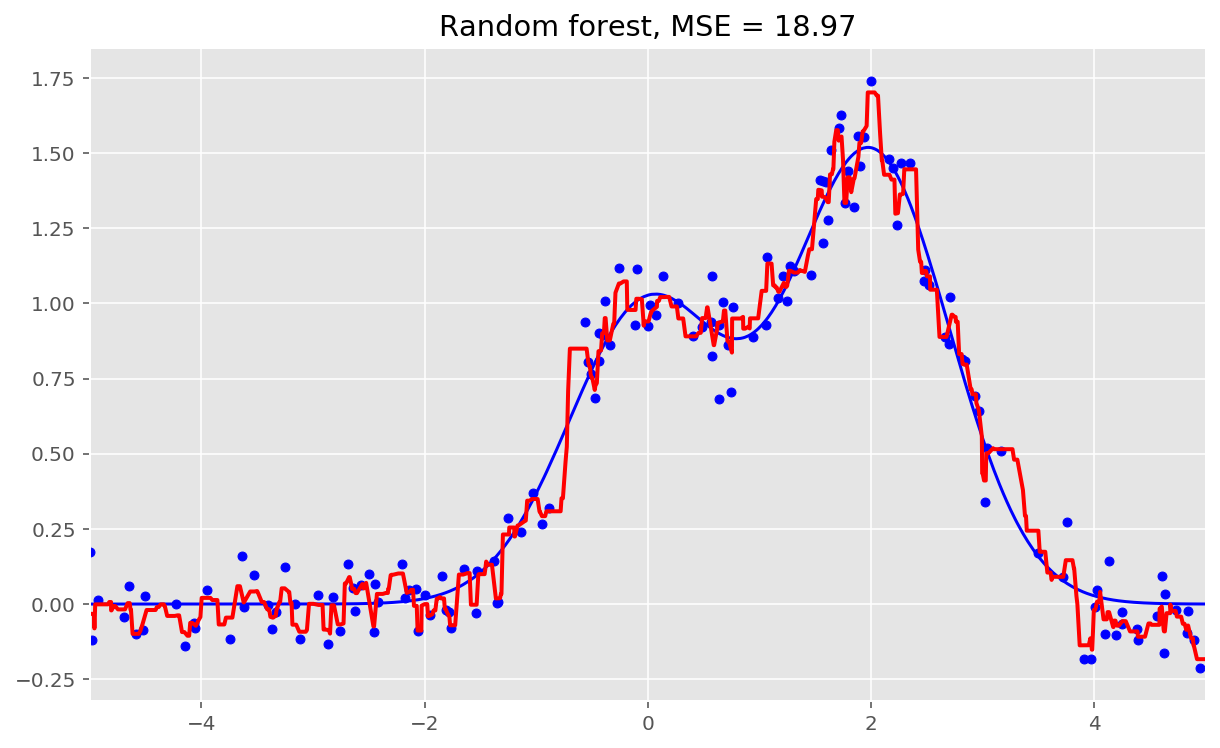As we can see from our graphs and the MSE values above, a random forest of 10 trees achieves a better result than a single decision tree and is comparable to bagging with 10 trees. The main difference between random forests and bagging is that, in a random forest, the best feature for a split is selected from a random subset of the available features while, in bagging, all features are considered for the next best split.

We can also look at the advantages of random forests and bagging in classification problems:

In :
np.random.seed(42)
X, y = make_circles(n_samples=500, factor=0.1, noise=0.35, random_state=42)
X_train_circles, X_test_circles, y_train_circles, y_test_circles = \
train_test_split(X, y, test_size=0.2)

dtree = DecisionTreeClassifier(random_state=42)
dtree.fit(X_train_circles, y_train_circles)

x_range = np.linspace(X.min(), X.max(), 100)
xx1, xx2 = np.meshgrid(x_range, x_range)
y_hat = dtree.predict(np.c_[xx1.ravel(), xx2.ravel()])
y_hat = y_hat.reshape(xx1.shape)
plt.contourf(xx1, xx2, y_hat, alpha=0.2)
plt.scatter(X[:,0], X[:,1], c=y, cmap='viridis', alpha=.7)
plt.title("Decision tree")
plt.show()

b_dtree = BaggingClassifier(DecisionTreeClassifier(),
n_estimators=300, random_state=42)
b_dtree.fit(X_train_circles, y_train_circles)

x_range = np.linspace(X.min(), X.max(), 100)
xx1, xx2 = np.meshgrid(x_range, x_range)
y_hat = b_dtree.predict(np.c_[xx1.ravel(), xx2.ravel()])
y_hat = y_hat.reshape(xx1.shape)
plt.contourf(xx1, xx2, y_hat, alpha=0.2)
plt.scatter(X[:,0], X[:,1], c=y, cmap='viridis', alpha=.7)
plt.title("Bagging (decision trees)")
plt.show()

rf = RandomForestClassifier(n_estimators=300, random_state=42)
rf.fit(X_train_circles, y_train_circles)

x_range = np.linspace(X.min(), X.max(), 100)
xx1, xx2 = np.meshgrid(x_range, x_range)
y_hat = rf.predict(np.c_[xx1.ravel(), xx2.ravel()])
y_hat = y_hat.reshape(xx1.shape)
plt.contourf(xx1, xx2, y_hat, alpha=0.2)
plt.scatter(X[:,0], X[:,1], c=y, cmap='viridis', alpha=.7)
plt.title("Random forest")
plt.show()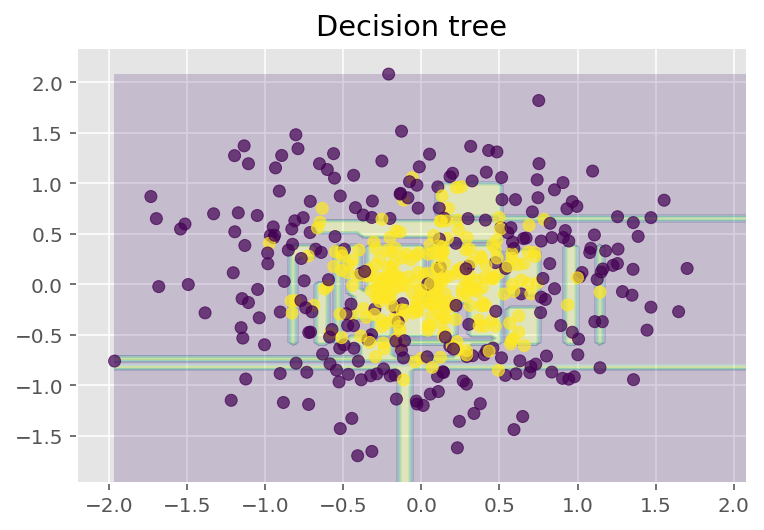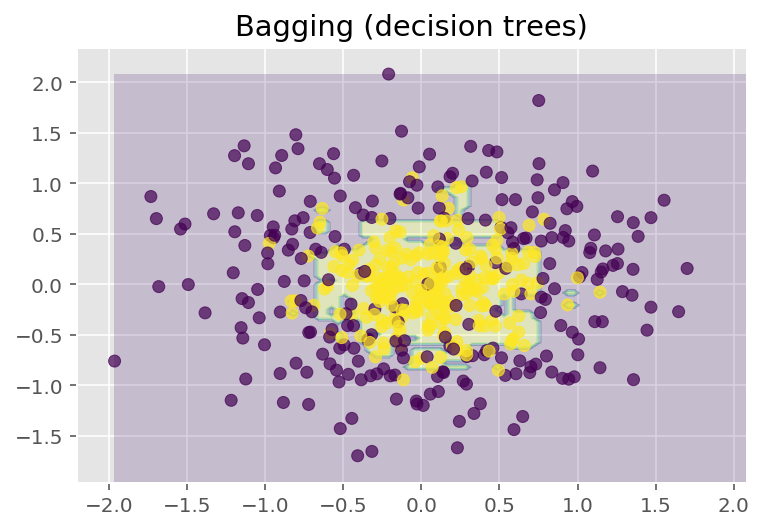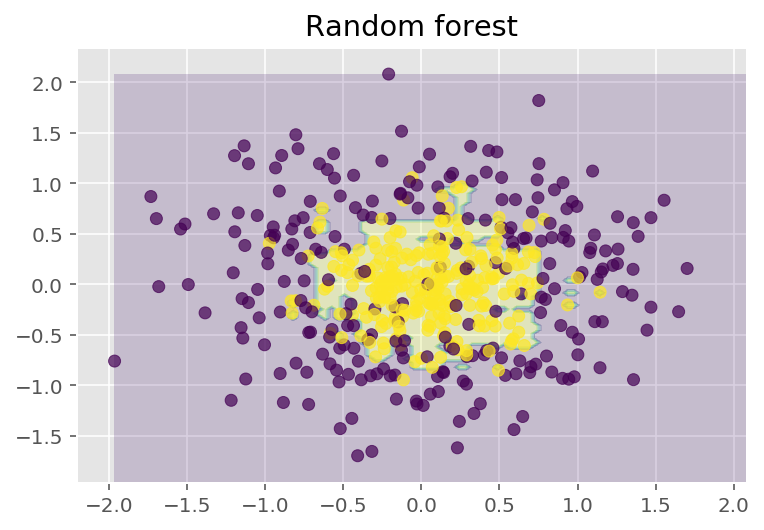The figures above show that the decision boundary of the decision tree is quite jagged and has a lot of acute angles that suggest overfitting and a weak ability to generalize. We would have trouble making reliable predictions on new test data. In contrast, the bagging algorithm has a rather smooth boundary and has no obvious signs of overfitting.

Now, let's investigate some parameters which can help us increase the model accuracy.

## 3. Parameters¶

The scikit-learn library implements random forests by providing two estimators: RandomForestClassifier and RandomForestRegressor.

The full list of random forest parameters for regression is shown below:

• n_estimators — the number of trees in the forest (default = 10)
• criterion — the function used to measure the quality of a split. Supported criteria are “mse” for the mean squared error, which is equal to variance reduction as feature selection criterion, and “mae” for the mean absolute error (default = "mse")
• max_features — the number of features to consider when looking for the best split. You can specify the number or percentage of features, or choose from the available values: "auto" (all features), "sqrt", "log2". (default = "auto")
• max_depth — the maximum depth of the tree (default means that nodes are expanded until all leaves are pure or until all leaves contain less than min_samples_split samples)
• min_samples_split — the minimum number of samples required to split an internal node. Can be specified as the number or as a percentage of a total number of samples (default = 2)
• min_samples_leaf — the minimum number of samples required at a leaf node(default = 1)
• min_weight_fraction_leaf — the minimum weighted fraction of the sum total of weights (of all the input samples) required to be at a leaf node. Samples have equal weight when sample_weight is not provided (default = 0)
• max_leaf_nodes — the maximum number of leaves (default = no restrictions)
• min_impurity_split — threshold for early stopping in tree growth. A node will split if its impurity is above the threshold, otherwise it is a leaf (default = 1е-7)
• bootstrap — whether bootstrap samples are used when building trees(default = True)
• oob_score — whether to use out-of-bag samples to estimate the R^2 on unseen data (default = False)
• n_jobs — the number of jobs to run in parallel for both fit and predict. If -1, then the number of jobs is set to the number of cores (default = 1)
• random_state — if int, random_state is the seed used by the random number generator; if RandomState instance, random_state is the random number generator; if None, the random number generator is the RandomState instance used by np.random (default = None)
• verbose — controls the verbosity of the tree building process (default = 0)
• warm_start — when set to True, reuse the solution of the previous call to fit and add more estimators to the ensemble, otherwise, just fit a whole new forest (default = False)

In case of classification, parameters are mostly the same. Only the following differ for RandomForestClassifier as compared to RandomForestRegressor:

• criterion — the function used to measure the quality of a split. Supported criteria are “gini” for the Gini impurity and “entropy” for the information gain. Note: this parameter is tree-specific (default = "gini")
• class_weight — the weight of each class (by default all weights equal to 1, but you can create a dictionary with weights or specify it as "balanced" - uses the values of classes to automatically adjust weights inversely proportional to class frequencies in the input data or as "balanced_subsample" - the same as “balanced” except that weights are computed based on the bootstrap sample for every tree grown)

Below are the parameters which we need to pay attention to when we are building a new model:

• n_estimators — the number of trees in the forest;
• criterion — the function used to measure the quality of a split;
• max_features — the number of features to consider when looking for the best split;
• min_samples_leaf — the minimum number of samples required to be at a leaf node;
• max_depth — the maximum depth of the tree.

#### Practice with random forests in a real problem¶

In this example we will look at predicting customer churn. This is a classification problem, so we will use accuracy for model evaluation.

First, let's build a simple classifier which we will use as a baseline. For the sake of simplicity, we will use only numeric features.

In :
import pandas as pd
from sklearn.model_selection import cross_val_score, StratifiedKFold, GridSearchCV
from sklearn.metrics import accuracy_score

# Choose the numeric features
cols = []
for i in df.columns:
if (df[i].dtype == "float64") or (df[i].dtype == 'int64'):
cols.append(i)

# Divide the dataset into the input and target
X, y = df[cols].copy(), np.asarray(df["Churn"],dtype='int8')

# Initialize a stratified split of our dataset for the validation process
skf = StratifiedKFold(n_splits=5, shuffle=True, random_state=42)

# Initialize the classifier with the default parameters
rfc = RandomForestClassifier(random_state=42, n_jobs=-1)

# Train it on the training set
results = cross_val_score(rfc, X, y, cv=skf)

# Evaluate the accuracy on the test set
print("CV accuracy score: {:.2f}%".format(results.mean() * 100))

CV accuracy score: 91.18%


We have accuracy equal to 91.18%. Now, let's try to improve this result, and take a look at the behavior of the learning curves when we change the basic parameters.

In :
# Initialize the validation
skf = StratifiedKFold(n_splits=5, shuffle=True, random_state=42)

# Create lists to save the values of accuracy on training and test sets
train_acc = []
test_acc = []
temp_train_acc = []
temp_test_acc = []
trees_grid = [5, 10, 15, 20, 30, 50, 75, 100]

for ntrees in trees_grid:
rfc = RandomForestClassifier(n_estimators=ntrees, random_state=42, n_jobs=-1)
temp_train_acc = []
temp_test_acc = []
for train_index, test_index in skf.split(X, y):
X_train, X_test = X.iloc[train_index], X.iloc[test_index]
y_train, y_test = y[train_index], y[test_index]
rfc.fit(X_train, y_train)
temp_train_acc.append(rfc.score(X_train, y_train))
temp_test_acc.append(rfc.score(X_test, y_test))
train_acc.append(temp_train_acc)
test_acc.append(temp_test_acc)

train_acc, test_acc = np.asarray(train_acc), np.asarray(test_acc)
print("Best CV accuracy is {:.2f}% with {} trees".format(max(test_acc.mean(axis=1))*100,
trees_grid[np.argmax(test_acc.mean(axis=1))]))

Best CV accuracy is 92.41% with 100 trees

In :
plt.style.use('ggplot')

fig, ax = plt.subplots(figsize=(8, 4))
ax.plot(trees_grid, train_acc.mean(axis=1), alpha=0.5, color='blue', label='train')
ax.plot(trees_grid, test_acc.mean(axis=1), alpha=0.5, color='red', label='cv')
ax.fill_between(trees_grid, test_acc.mean(axis=1) - test_acc.std(axis=1),
test_acc.mean(axis=1) + test_acc.std(axis=1), color='#888888', alpha=0.4)
ax.fill_between(trees_grid, test_acc.mean(axis=1) - 2*test_acc.std(axis=1),
test_acc.mean(axis=1) + 2*test_acc.std(axis=1), color='#888888', alpha=0.2)
ax.legend(loc='best')
ax.set_ylim([0.88,1.02])
ax.set_ylabel("Accuracy")
ax.set_xlabel("N_estimators");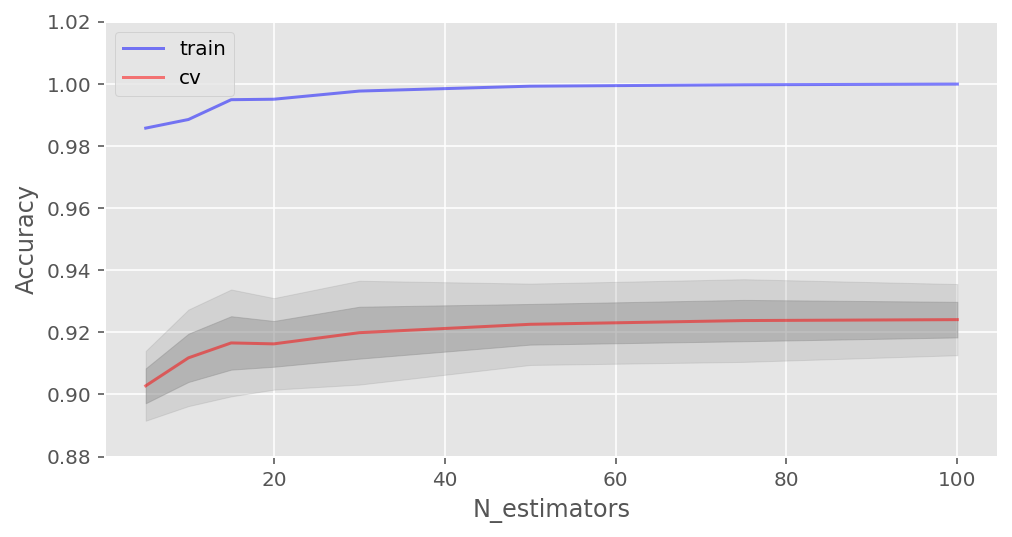As you can see, when a certain number of trees is reached, our accuracy on the test set is very close to the asymptote. You can decide by yourself which value would be the optimal number of trees for your problem.

The figures also show that we achieved 100% accuracy on the training set, which tells us that we overfit. In order to avoid overfitting, we need to add regularization parameters to our model.

We will start with the maximum depth of trees max_depth and fix the number of trees at 100:

In :
# Create lists to save accuracy values on the training and test sets
train_acc = []
test_acc = []
temp_train_acc = []
temp_test_acc = []
max_depth_grid = [3, 5, 7, 9, 11, 13, 15, 17, 20, 22, 24]

for max_depth in max_depth_grid:
rfc = RandomForestClassifier(n_estimators=100, random_state=42, n_jobs=-1, max_depth=max_depth)
temp_train_acc = []
temp_test_acc = []
for train_index, test_index in skf.split(X, y):
X_train, X_test = X.iloc[train_index], X.iloc[test_index]
y_train, y_test = y[train_index], y[test_index]
rfc.fit(X_train, y_train)
temp_train_acc.append(rfc.score(X_train, y_train))
temp_test_acc.append(rfc.score(X_test, y_test))
train_acc.append(temp_train_acc)
test_acc.append(temp_test_acc)

train_acc, test_acc = np.asarray(train_acc), np.asarray(test_acc)
print("Best CV accuracy is {:.2f}% with {} max_depth".format(max(test_acc.mean(axis=1))*100,
max_depth_grid[np.argmax(test_acc.mean(axis=1))]))

fig, ax = plt.subplots(figsize=(8, 4))
ax.plot(max_depth_grid, train_acc.mean(axis=1), alpha=0.5, color='blue', label='train')
ax.plot(max_depth_grid, test_acc.mean(axis=1), alpha=0.5, color='red', label='cv')
ax.fill_between(max_depth_grid, test_acc.mean(axis=1) - test_acc.std(axis=1),
test_acc.mean(axis=1) + test_acc.std(axis=1), color='#888888', alpha=0.4)
ax.fill_between(max_depth_grid, test_acc.mean(axis=1) - 2*test_acc.std(axis=1),
test_acc.mean(axis=1) + 2*test_acc.std(axis=1), color='#888888', alpha=0.2)
ax.legend(loc='best')
ax.set_ylim([0.88,1.02])
ax.set_ylabel("Accuracy")
ax.set_xlabel("Max_depth");

Best CV accuracy is 92.65% with 15 max_depth